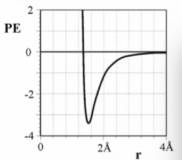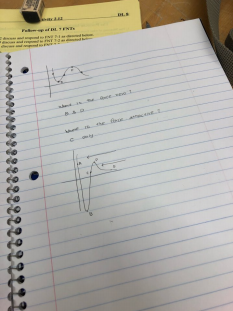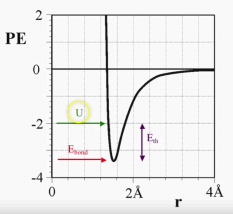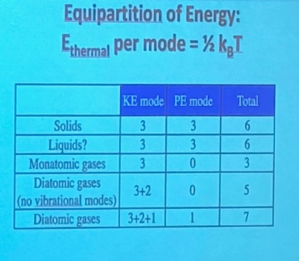×
Get Full Access to UCD - PHY 7 - Study Guide - Final
Get Full Access to UCD - PHY 7 - Study Guide - Final

×

UCD / Physician Assistant / PHY 7 / What is the meaning of gravitational potential energy?

# What is the meaning of gravitational potential energy? Description

##### Description: Study Guide
9 Pages 137 Views 2 Unlocks
Reviews

Lecture 1

## What is the meaning of gravitational potential energy?Conservation of Energy (1st law):

-energy is not lost or created

-transformed into thermal energy

Kinetic Energy:

1/2 mv^2

-marker: speed

-has to be positive

Gravitational Potential Energy:

mgh

-marker: height

-can be negative..depending on where we set the 0 potential energy

Spring Potential Energy:

1/2 kd^2

-marker: spring length away from equilibrium

at equilibrium: highest kinetic energy

not at equilibrium: high ends of potential energy  We also discuss several other topics like What is the dynamic nature of chemical equilibria?

Force

-goes toward lower PE

-open: W = change of KE = -mg change x

## What is the meaning of spring potential energy?-closed:

Heat (Q):

-transfer of energy from higher temp to lower temp

-no transfer : thermal equilibrium : same temp

usually Q= Eth + Eb = m delta H + mc change of T

Work (W):

-energy other than heat …and involves a force

W = F x change of distance

Bond Energy:

m delta H

-marker: amount of phase to the right

Thermal Energy:

mc change of T

-marker: temperature

Heat Capacity - associated with Ethermal

Understand the equation:

Q (heat Joules) = C (heat capacity) * change of T = change of Ethermal C (heat capacity) = m(mass) c (specific heat)

## Bond energy refers to what?change of Eth = mc *change of T Don't forget about the age old question of What is the meaning of continuous tone photography?

Heats of Vaporization and Melting - associated with Ebond Understand the equation:

Q (heat Joules) = delta H (heat vap/melt) = change of Ebond

-> mass * delta H (heat vap/melt) or mole * delta H (heat vap/melt)

*To find energy : need mass of the objects, initial / final temperatures, specific heat (given on table) If you want to learn more check out What is the purpose of public opinion?

*Associate each bubble with different energy …specific heat is different

Internal Energy (U):

E total = U = Ebond + E th = Q +W (open system)

Systems:

Closed:

-conservation of energy

Change in Total Energy = 0

Open

Change in Total Energy = change Eb + change Eth … = energy added - energy removed = Q + W

Three Phase Diagram

y axis: Temperature (K,C)

/ - slope represent change of temperature , same phase

— - flat line represent change of phase, same temperature

*read carefully the initial points and predict final by corresponding the temperature / energy with the lines

Energy Interaction Model

open system: dashed circle

closed system: solid circle

ONE “bubble”

-represent one phase change  If you want to learn more check out What is the process through which evolution occurs?

-initial: solid final: gas …2 bubbles: Eb increase 1 s-> l , Eb increase1 l->g -represent temperature change (separate at each melting or boiling point: ice, liquid, vapor have different specific heat)

-initial: -19C final: 20C …2 bubbles: Eth increase -19C to 0C , Eth increase 0C to 20C Equation:

closed system: total change of energy = 0

open system: total change of energy = change of thermal energy + change of bond energy

Exceptions to the three phase diagram

-can have extended phase at certain temperature.

ex) heat pack - supercooling: solid at room temperature We also discuss several other topics like What program is believed to have first been used in 1974 by bell labs technicians?

*focus on what is different and extend or shorten the line... and then see if it will follow the normal three phase diagram Don't forget about the age old question of How does an adaptation affect the fitness of a species?

closed system: energy doesn’t change

open system: energy can possible go back to the normal three phase diagram or along the “extended” phase

3) Chemical Concept

-can apply the energy diagram for chemical equations

-bonds breaking =energy added= bond energy increases; bonds forming = energy released = bond energy decreases

Equation: [reactants] -> [products]

-bubble: object - Eb increase/decrease - mass of object decrease/increase -reactants: breaking apart , Eb increase ; product: forming, Eb decreases -total change of energy = +/- moles of Eb energy

Concepts:

-Heat is not contained in an object…it is the amount of energy transferred between objects

-Thermal Equilibrium: the final temperature will between the initial temperatures of the objects

Main:

1. Identify the changes in the system

-temperature/thermal energy increase or decrease

-bond energy increase or decrease

2. Separate them correctly

-temperature should be separated based on melting/boiling point  -phase should be separated based on the phases

3. Associate the correct equation to the bubbles

-thermal energy: uses heat capacity/ specific heat

-bond energy: uses heat of melting or heat of vaporization

4. Sum up the energy to get total energy

-thermal increase = + energy , thermal decrease = - energy

-bond increase = + energy, bond decrease = - energy

Graphlarge r : balls are attracted to each other ..since PE decrease as r decrease small r : balls are repelled …since PE decreases when r increases (by a little ) * typical chemical atom

Force is zero at the extreme endsA: repulsive

B: no force

C: attractive

D: no force

E almost no interactionE total = Internal Energy

PE min = E bond (well depth)

KE increase = start from PE min and reflect it up …. within the range the two E total points

Bounded atoms:

E tot < 0

KE (r min and r max)

Unbounded atoms:

E tot >0

KE (r min..max: asymptote)

Typical Atom Shape:

12 bonds

6Na E

Modes:bond energy (E bond) = number of bonds (epsilon well depth)

number of bonds = number of atoms (Na) x number of nearest neighbor per atom / 2 E bond =( number of atom x number of neighbor per atom / 2 ) e

higher E bond more stable

DL 11

realistic of bond energy greater than 6 due to the influence of next next neighbors

PE: shows a point

change of PE: shows the r min and r max

PE average : shows the overall

for oscillating springs: PE average =KE average

Combining microscopic and macroscopic energy

Etot=KE+PE =Ebond+Ethermal

temperatures proportion to Ethermal

starting PE = Ebond

E bond stays the same

E thermal increases as temperature increases

PE and KE base on E total

Liquid/Solid Equation:

Eth associate with oscillating -> oscillating means equal KE average and PE average -> KE total and PE total changes

KEtotal = 0.5Eth

PE total = E bond + 0.5 Eth

E total = 0.5 Eth + 0.5 Eth + E bond

Spring model: associate with PE = 3

KE/PE: 3

6 modes

Gas Equation =

monoatomic:

KE = Eth

PE = 0

*some has PE some doesn’t

PE associated with vibrational meaning only diatomic will have PE

Thermal Energy:

greater when temperature is higher, diatomic, mole is greater (1 mole = 1mode, 2 mole = 2 mode) , gas

DL 12

-larger diameter larger r0

-smaller well depth easier to break

graph to see that there is 6 modes

gas / liquid/ solid :

E thermal = total # of modes x 1/2 Kb T = slope

Week 6

degrees of freedom = number of ways to move

mode = number of ways to have energy

KE = 1/2 mv x, y, z ^2

PE = 1/2 kd x, y, z^2

Week 7

*4 atoms in square

r0 , r0 , sqrt (r0 ^2 + r0^2) = sqrt (2 r0^2) -> sqrt(2) r0

For non linear complex molecules:

KE = 3N….3 Trans 3 Rot 3N-6 Vib

PE = 3N-6

T number of modes: 6(N-1)

FNT 14

Interpreting Cv/R vs T graph gas

Cv (molar) = number of mode / 2 x R

Cv/R = number of modes/2

info:

-vibrational mode increases as temperature increases

-linear shape molecules 2 rotational mode , non linear shape molecules 3 rotational mode -smaller gap between ground and excited state = more energy per mode

Comparing two molecules

-rotational: look at atomic number (mono, dia, poly)

-look at translational (usually always 3)

-active mode, gap between excited/ground: can look at graph

-translational speed: look at weight

Thermodynamics:

Internal Energy: Eth + Eb

Equation: change of U = Q + W

State Functions:

-only depend on the initial and final

-ex) Eth, Ebond, U, P, T, KE, PE

Process depend:

-process can change the values

-ex) Q, W

-no finial and initial so don’t have “change of”

Work:

-area under PV

-opposite of V…as V increases Work decrease (leave system..work done by system) / V decreases Work increases (enter system…work done on system)

Heat:

-can be defined as Q = Eth when there is no phase change

Heat Capacity:

constant volume:

adding heat = KE increase = T increase =P increase = U increase

graph: UT (positive linear), PV(vertical line..no work, since volume is constant)

DL 15

Isothermal:

-constant temperature

U = 0 (U=Q=Eth) , (Q = m cp T)

Isochoric:

-constant volume

W = 0

Isobaric

-constant pressure

Q, W, U will be affected

-no heat transfer

Q = 0

No Work transfer:

W= 0 (W= - integral P dv)

PV graph:

-line depends on T

-double T …more difference in the beginning…less changes when volume is big UT graph:

-slope shows the heat capacity

Thermodynamic models:

Enthalpy vs Temperature diagram

-different process …but with state functions can find change in H

-change of H (specific) = n Cp T + change of Hap

*use kJ/mol….J/K mol

PV diagrams:

-work: area under the curve

-if going to left…work = neg

-if going to right…work =pos

-if given: mode, mole can find U = 1/2 mode n RT

-T = PV/R

Formulas:

PV= nRT

(U) Eth = 1/2 Kb Na n (modes) change of T

Q= change of U - W ….change of U = Q + W

Cv/R = number of modes/2

Cp = Q /change of T = (change of Eth - P change of V)/ T = Cv + P change of V / T = n Cv molar + nR

Page ExpiredIt looks like your free minutes have expired! Lucky for you we have all the content you need, just sign up here